# Generic Programming¶

I find OOP methodologically wrong. It starts with classes. It is as if mathematicians would start with axioms. You do not start with axioms - you start with proofs. Only when you have found a bunch of related proofs, can you come up with axioms. You end with axioms. The same thing is true in programming: you have to start with interesting algorithms. Only when you understand them well, can you come up with an interface that will let them work. – Alexander Stepanov

## Overview¶

In this lecture we delve more deeply into the structure of Julia, and in particular into

• abstract and concrete types
• the type tree
• designing and using generic interfaces
• the role of generic interfaces in Julia performance

• form a “mental model” of the Julia language
• design code that matches the “white-board” mathematics
• create code that can use (and be used by) a variety of other packages
• write “well organized” Julia code that’s easy to read, modify, maintain and debug
• improve the speed at which your code runs

(Special thank you to Jeffrey Sarnoff)

### Generic Programming is an Attitude¶

From Mathematics to Generic Programming [SR14]

Generic programming is an approach to programming that focuses on designing algorithms and data structures so that they work in the most general setting without loss of efficiency… Generic programming is more of an attitude toward programming than a particular set of tools.

In that sense, it is important to think of generic programming as an interactive approach to uncover generality without compromising performance rather than as a set of rules.

As we will see, the core approach is to treat data structures and algorithms as loosely coupled, and is in direct contrast to the is-a approach of object-oriented programming.

This lecture has the dual role of giving an introduction into the design of generic algorithms and describing how Julia helps make that possible.

### Setup¶

In :
using InstantiateFromURL
# optionally add arguments to force installation: instantiate = true, precompile = true
github_project("QuantEcon/quantecon-notebooks-julia", version = "0.8.0")

In :
using LinearAlgebra, Statistics
using Distributions, Plots, QuadGK, Polynomials, Interpolations
gr(fmt = :png);


## Exploring Type Trees¶

The connection between data structures and the algorithms which operate on them is handled by the type system.

Concrete types (i.e., Float64 or Array{Float64, 2}) are the data structures we apply an algorithm to, and the abstract types (e.g. the corresponding Number and AbstractArray) provide the mapping between a set of related data structures and algorithms.

In :
using Distributions
x = 1
y = Normal()
z = "foo"
@show x, y, z
@show typeof(x), typeof(y), typeof(z)
@show supertype(typeof(x))

# pipe operator, |>, is is equivalent
@show typeof(x) |> supertype
@show supertype(typeof(y))
@show typeof(z) |> supertype
@show typeof(x) <: Any;

(x, y, z) = (1, Normal{Float64}(μ=0.0, σ=1.0), "foo")
(typeof(x), typeof(y), typeof(z)) = (Int64, Normal{Float64}, String)
supertype(typeof(x)) = Signed
typeof(x) |> supertype = Signed
supertype(typeof(y)) = Distribution{Univariate,Continuous}
typeof(z) |> supertype = AbstractString
typeof(x) <: Any = true


Beyond the typeof and supertype functions, a few other useful tools for analyzing the tree of types are discussed in the introduction to types lecture

In :
using Base: show_supertypes # import the function from the Base package

show_supertypes(Int64)

Int64 <: Signed <: Integer <: Real <: Number <: Any
In :
subtypes(Integer)

Out:
4-element Array{Any,1}:
Bool
GeometryTypes.OffsetInteger
Signed
Unsigned

Using the subtypes function, we can write an algorithm to traverse the type tree below any time t – with the confidence that all types support subtypes

In :
#  from https://github.com/JuliaLang/julia/issues/24741
function subtypetree(t, level=1, indent=4)
if level == 1
println(t)
end
for s in subtypes(t)
println(join(fill(" ", level * indent)) * string(s))  # print type
subtypetree(s, level+1, indent)  # recursively print the next type, indenting
end
end

Out:
subtypetree (generic function with 3 methods)

Applying this to Number, we see the tree of types currently loaded

In :
subtypetree(Number) # warning: do not use this function on Any!

Number
Complex
Real
AbstractFloat
BigFloat
Float16
Float32
Float64
AbstractIrrational
Irrational
FixedPointNumbers.FixedPoint
FixedPointNumbers.Fixed
FixedPointNumbers.Normed
Integer
Bool
GeometryTypes.OffsetInteger
Signed
BigInt
Int128
Int16
Int32
Int64
Int8
Unsigned
UInt128
UInt16
UInt32
UInt64
UInt8
Rational
Ratios.SimpleRatio
StatsBase.TestStat


For the most part, all of the “leaves” will be concrete types.

### Any¶

At the root of all types is Any

There are a few functions that work in the “most generalized” context: usable with anything that you can construct or access from other packages.

We have already called typeof, show and supertype – which will apply to a custom struct type since MyType <: Any

In :
# custom type
struct MyType
a::Float64
end

myval = MyType(2.0)
@show myval
@show typeof(myval)
@show supertype(typeof(myval))
@show typeof(myval) <: Any;

myval = MyType(2.0)
typeof(myval) = MyType
supertype(typeof(myval)) = Any
typeof(myval) <: Any = true


Here we see another example of generic programming: every type <: Any supports the @show macro, which in turn, relies on the show function.

The @show macro (1) prints the expression as a string; (2) evaluates the expression; and (3) calls the show function on the returned values.

To see this with built-in types

In :
x = [1, 2]
show(x)

[1, 2]

The Any type is useful, because it provides a fall-back implementation for a variety of functions.

Hence, calling show on our custom type dispatches to the fallback function

In :
myval = MyType(2.0)
show(myval)

MyType(2.0)

The default fallback implementation used by Julia would be roughly equivalent to

function show(io::IO, x)
str = string(x)
print(io, str)
end


To implement a specialized implementation of the show function for our type, rather than using this fallback

In :
import Base.show  # to extend an existing function

function show(io::IO, x::MyType)
str = "(MyType.a = $(x.a))" # custom display print(io, str) end show(myval) # it creates an IO value first and then calls the above show  (MyType.a = 2.0) At that point, we can use the @show macro, which in turn calls show In : @show myval;  myval = (MyType.a = 2.0)  Here we see another example of generic programming: any type with a show function works with @show. Layering of functions (e.g. @show calling show) with a “fallback” implementation makes it possible for new types to be designed and only specialized where necessary. ### Unlearning Object Oriented (OO) Programming (Advanced)¶ See Types for more on OO vs. generic types. If you have never used programming languages such as C++, Java, and Python, then the type hierarchies above may seem unfamiliar and abstract. In that case, keep an open mind that this discussion of abstract concepts will have practical consequences, but there is no need to read this section. Otherwise, if you have used object-oriented programming (OOP) in those languages, then some of the concepts in these lecture notes will appear familiar. Don’t be fooled! The superficial similarity can lead to misuse: types are not classes with poor encapsulation, and methods are not the equivalent to member functions with the order of arguments swapped. In particular, previous OO knowledge often leads people to write Julia code such as In : # BAD! Replicating an OO design in Julia mutable struct MyModel a::Float64 b::Float64 algorithmcalculation::Float64 MyModel(a, b) = new(a, b, 0.0) # an inner constructor end function myalgorithm!(m::MyModel, x) m.algorithmcalculation = m.a + m.b + x # some algorithm end function set_a!(m::MyModel, a) m.a = a end m = MyModel(2.0, 3.0) x = 0.1 set_a!(m, 4.1) myalgorithm!(m, x) @show m.algorithmcalculation;  m.algorithmcalculation = 7.199999999999999  You may think to yourself that the above code is similar to OO, except that you • reverse the first argument, i.e., myalgorithm!(m, x) instead of the object-oriented m.myalgorithm!(x) • cannot control encapsulation of the fields a, b, but you can add getter/setters like set_a • do not have concrete inheritance While this sort of programming is possible, it is (verbosely) missing the point of Julia and the power of generic programming. When programming in Julia • there is no encapsulation and most custom types you create will be immutable. • Polymorphism is achieved without anything resembling OOP inheritance. • Abstraction is implemented by keeping the data and algorithms that operate on them as orthogonal as possible – in direct contrast to OOP’s association of algorithms and methods directly with a type in a tree. • The supertypes in Julia are simply used for selecting which specialized algorithm to use (i.e., part of generic polymorphism) and have nothing to do with OO inheritance. • The looseness that accompanies keeping algorithms and data structures as orthogonal as possible makes it easier to discover commonality in the design. #### Iterative Design of Abstractions¶ As its essence, the design of generic software is that you will start with creating algorithms which are largely orthogonal to concrete types. In the process, you will discover commonality which leads to abstract types with informally defined functions operating on them. Given the abstract types and commonality, you then refine the algorithms as they are more limited or more general than you initially thought. This approach is in direct contrast to object-oriented design and analysis (OOAD). With that, where you specify a taxonomies of types, add operations to those types, and then move down to various levels of specialization (where algorithms are embedded at points within the taxonomy, and potentially specialized with inheritance). In the examples that follow, we will show for exposition the hierarchy of types and the algorithms operating on them, but the reality is that the algorithms are often designed first, and the abstact types came later. ## Distributions¶ First, consider working with “distributions”. Algorithms using distributions might (1) draw random numbers for Monte-Carlo methods; and (2) calculate the pdf or cdf – if it is defined. The process of using concrete distributions in these sorts of applications led to the creation of the Distributions.jl package. Let’s examine the tree of types for a Normal distribution In : using Distributions d1 = Normal(1.0, 2.0) # an example type to explore @show d1 show_supertypes(typeof(d1))  d1 = Normal{Float64}(μ=1.0, σ=2.0) Normal{Float64} <: Distribution{Univariate,Continuous} <: Sampleable{Univariate,Continuous} <: Any The Sampleable{Univariate,Continuous} type has a limited number of functions, chiefly the ability to draw a random number In : @show rand(d1);  rand(d1) = 2.1661846554373643  The purpose of that abstract type is to provide an interface for drawing from a variety of distributions, some of which may not have a well-defined predefined pdf. If you were writing a function to simulate a stochastic process with arbitrary iid shocks, where you did not need to assume an existing pdf etc., this is a natural candidate. For example, to simulate$ x_{t+1} = a x_t + b \epsilon_{t+1} $where$ \epsilon \sim D $for some$ D $, which allows drawing random values. In : function simulateprocess(x₀; a = 1.0, b = 1.0, N = 5, d::Sampleable{Univariate,Continuous}) x = zeros(typeof(x₀), N+1) # preallocate vector, careful on the type x = x₀ for t in 2:N+1 x[t] = a * x[t-1] + b * rand(d) # draw end return x end @show simulateprocess(0.0, d=Normal(0.2, 2.0));  simulateprocess(0.0, d = Normal(0.2, 2.0)) = [0.0, 0.855506812621363, -0.7949707393207366, 2.1284021422981976, 1.577107690765248, 3.7524511274361445]  The Sampleable{Univariate,Continuous} and, especially, the Sampleable{Multivariate,Continuous} abstract types are useful generic interfaces for Monte-Carlo and Bayesian methods. Moving down the tree, the Distributions{Univariate, Continuous} abstract type has other functions we can use for generic algorithms operating on distributions. These match the mathematics, such as pdf, cdf, quantile, support, minimum, maximum, etc. In : d1 = Normal(1.0, 2.0) d2 = Exponential(0.1) @show d1 @show d2 @show supertype(typeof(d1)) @show supertype(typeof(d2)) @show pdf(d1, 0.1) @show pdf(d2, 0.1) @show cdf(d1, 0.1) @show cdf(d2, 0.1) @show support(d1) @show support(d2) @show minimum(d1) @show minimum(d2) @show maximum(d1) @show maximum(d2);  d1 = Normal{Float64}(μ=1.0, σ=2.0) d2 = Exponential{Float64}(θ=0.1) supertype(typeof(d1)) = Distribution{Univariate,Continuous} supertype(typeof(d2)) = Distribution{Univariate,Continuous} pdf(d1, 0.1) = 0.18026348123082397 pdf(d2, 0.1) = 3.6787944117144233 cdf(d1, 0.1) = 0.32635522028792 cdf(d2, 0.1) = 0.6321205588285577 support(d1) = RealInterval(-Inf, Inf) support(d2) = RealInterval(0.0, Inf) minimum(d1) = -Inf minimum(d2) = 0.0 maximum(d1) = Inf maximum(d2) = Inf  You could create your own Distributions{Univariate, Continuous} type by implementing those functions – as is described in the documentation. If you fulfill all of the conditions of a particular interface, you can use algorithms from the present, past, and future that are written for the abstract Distributions{Univariate, Continuous} type. As an example, consider the StatsPlots package In : using StatsPlots d = Normal(2.0, 1.0) plot(d) # note no other arguments!  Out: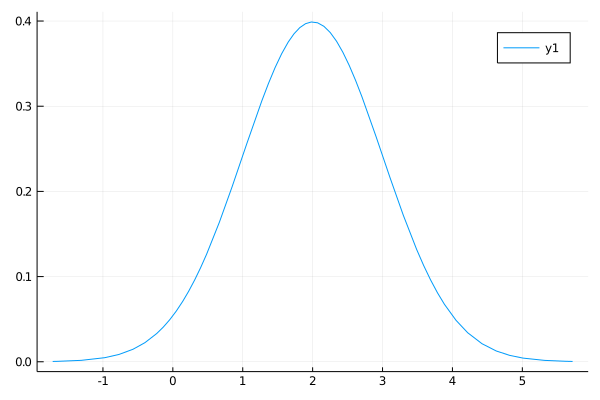Calling plot on any subtype of Distributions{Univariate, Continuous} displays the pdf and uses minimum and maximum to determine the range. Let’s create our own distribution type In : struct OurTruncatedExponential <: Distribution{Univariate,Continuous} α::Float64 xmax::Float64 end Distributions.pdf(d::OurTruncatedExponential, x) = d.α *exp(-d.α * x)/exp(-d.α * d.xmax) Distributions.minimum(d::OurTruncatedExponential) = 0 Distributions.maximum(d::OurTruncatedExponential) = d.xmax # ... more to have a complete type  To demonstrate this In : d = OurTruncatedExponential(1.0,2.0) @show minimum(d), maximum(d) @show support(d) # why does this work?  (minimum(d), maximum(d)) = (0, 2.0) support(d) = RealInterval(0.0, 2.0)  Out: RealInterval(0.0, 2.0) Curiously, you will note that the support function works, even though we did not provide one. This is another example of the power of multiple dispatch and generic programming. In the background, the Distributions.jl package has something like the following implemented Distributions.support(d::Distribution) = RealInterval(minimum(d), maximum(d))  Since OurTruncatedExponential <: Distribution, and we implemented minimum and maximum, calls to support get this implementation as a fallback. These functions are enough to use the StatsPlots.jl package In : plot(d) # uses the generic code!  Out: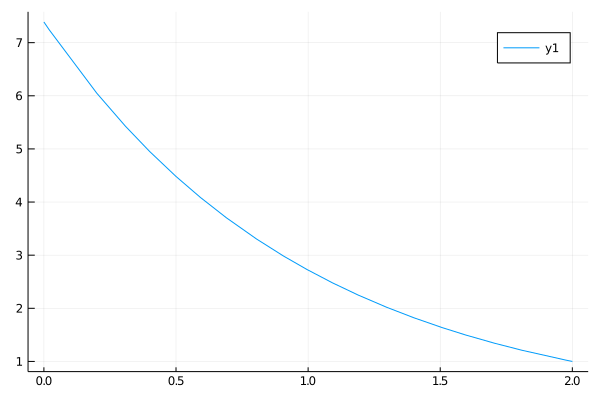A few things to point out • Even if it worked for StatsPlots, our implementation is incomplete, as we haven’t fulfilled all of the requirements of a Distribution. • We also did not implement the rand function, which means we are breaking the implicit contract of the Sampleable abstract type. • It turns out that there is a better way to do this precise thing already built into Distributions. In : d = Truncated(Exponential(0.1), 0.0, 2.0) @show typeof(d) plot(d)  typeof(d) = Truncated{Exponential{Float64},Continuous,Float64}  Out: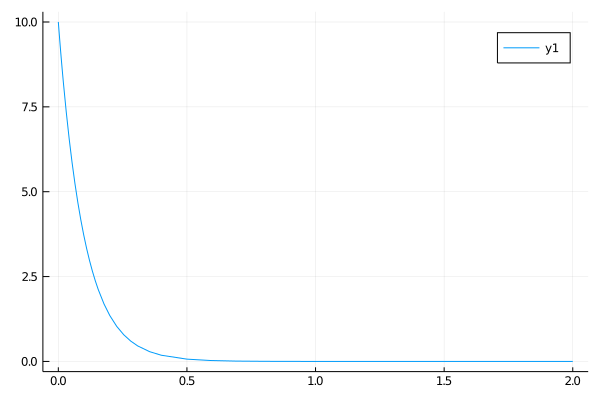This is the power of generic programming in general, and Julia in particular: you can combine and compose completely separate packages and code, as long as there is an agreement on abstract types and functions. ## Numbers and Algebraic Structures¶ Define two binary functions,$ + $and$ \cdot $, called addition and multiplication – although the operators can be applied to data structures much more abstract than a Real. In mathematics, a ring is a set with associated additive and multiplicative operators where • the additive operator is associative and commutative • the multiplicative operator is associative and distributive with respect to the additive operator • there is an additive identity element, denoted$ 0 $, such that$ a + 0 = a $for any$ a $in the set • there is an additive inverse of each element, denoted$ -a $, such that$ a + (-a) = 0 $• there is a multiplicative identity element, denoted$ 1 $, such that$ a \cdot 1 = a = 1 \cdot a $• a total or partial ordering is not required (i.e., there does not need to be any meaningful$ < $operator defined) • a multiplicative inverse is not required While this skips over some parts of the mathematical definition, this algebraic structure provides motivation for the abstract Number type in Julia • Remark: We use the term “motivation” because they are not formally connected and the mapping is imperfect. • The main difficulty when dealing with numbers that can be concretely created on a computer is that the requirement that the operators are closed in the set are difficult to ensure (e.g. floating points have finite numbers of bits of information). Let typeof(a) = typeof(b) = T <: Number, then under an informal definition of the generic interface for Number, the following must be defined • the additive operator: a + b • the multiplicative operator: a * b • an additive inverse operator: -a • an inverse operation for addition a - b = a + (-b) • an additive identity: zero(T) or zero(a) for convenience • a multiplicative identity: one(T) or one(a) for convenience The core of generic programming is that, given the knowledge that a value is of type Number, we can design algorithms using any of these functions and not concern ourselves with the particular concrete type. Furthermore, that generality in designing algorithms comes with no compromises on performance compared to carefully designed algorithms written for that particular type. To demonstrate this for a complex number, where Complex{Float64} <: Number In : a = 1.0 + 1.0im b = 0.0 + 2.0im @show typeof(a) @show typeof(a) <: Number @show a + b @show a * b @show -a @show a - b @show zero(a) @show one(a);  typeof(a) = Complex{Float64} typeof(a) <: Number = true a + b = 1.0 + 3.0im a * b = -2.0 + 2.0im -a = -1.0 - 1.0im a - b = 1.0 - 1.0im zero(a) = 0.0 + 0.0im one(a) = 1.0 + 0.0im  And for an arbitrary precision integer where BigInt <: Number (i.e., a different type than the Int64 you have worked with, but nevertheless a Number) In : a = BigInt(10) b = BigInt(4) @show typeof(a) @show typeof(a) <: Number @show a + b @show a * b @show -a @show a - b @show zero(a) @show one(a);  typeof(a) = BigInt typeof(a) <: Number = true a + b = 14 a * b = 40 -a = -10 a - b = 6 zero(a) = 0 one(a) = 1  ### Complex Numbers and Composition of Generic Functions¶ This allows us to showcase further how different generic packages compose – even if they are only loosely coupled through agreement on common generic interfaces. The Complex numbers require some sort of storage for their underlying real and imaginary parts, which is itself left generic. This data structure is defined to work with any type <: Number, and is parameterized (e.g. Complex{Float64} is a complex number storing the imaginary and real parts in Float64) In : x = 4.0 + 1.0im @show x, typeof(x) xbig = BigFloat(4.0) + 1.0im @show xbig, typeof(xbig);  (x, typeof(x)) = (4.0 + 1.0im, Complex{Float64}) (xbig, typeof(xbig)) = (4.0 + 1.0im, Complex{BigFloat})  The implementation of the Complex numbers use the underlying operations of storage type, so as long as +, * etc. are defined – as they should be for any Number – the complex operation can be defined In : @which +(x,x)  Out: +(z::Complex, w::Complex) in Base at complex.jl:275 Following that link, the implementation of + for complex numbers is +(z::Complex, w::Complex) = Complex(real(z) + real(w), imag(z) + imag(w))  real(z) and imag(z) returns the associated components of the complex number in the underlying storage type (e.g. Float64 or BigFloat). The rest of the function has been carefully written to use functions defined for any Number (e.g. + but not <, since it is not part of the generic number interface). To follow another example , look at the implementation of abs specialized for complex numbers In : @which abs(x)  Out: abs(z::Complex) in Base at complex.jl:264 The source is abs(z::Complex) = hypot(real(z), imag(z))  In this case, if you look at the generic function to get the hypotenuse, hypot, you will see that it has the function signature hypot(x::T, y::T) where T<:Number, and hence works for any Number. That function, in turn, relies on the underlying abs for the type of real(z). This would dispatch to the appropriate abs for the type In : @which abs(1.0)  Out: abs(x::Float64) in Base at float.jl:528 In : @which abs(BigFloat(1.0))  Out: abs(x::Real) in Base at number.jl:120 With implementations abs(x::Real) = ifelse(signbit(x), -x, x) abs(x::Float64) = abs_float(x)  For a Real number (which we will discuss in the next section) the fallback implementation calls a function signbit to determine if it should flip the sign of the number. The specialized version for Float64 <: Real calls a function called abs_float – which turns out to be a specialized implementation at the compiler level. While we have not completely dissected the tree of function calls, at the bottom of the tree you will end at the most optimized version of the function for the underlying datatype. Hopefully this showcases the power of generic programming: with a well-designed set of abstract types and functions, the code can both be highly general and composable and still use the most efficient implementation possible. ## Reals and Algebraic Structures¶ Thinking back to the mathematical motivation, a field is a ring with a few additional properties, among them • a multiplicative inverse:$ a^{-1} $• an inverse operation for multiplication:$ a / b = a \cdot b^{-1} $Furthermore, we will make it a total ordered field with • a total ordering binary operator:$ a < b $This type gives some motivation for the operations and properties of the Real type. Of course, Complex{Float64} <: Number but not Real – since the ordering is not defined for complex numbers in mathematics. These operations are implemented in any subtype of Real through • the multiplicative inverse: inv(a) • the multiplicative inverse operation: a / b = a * inv(b) • an ordering a < b We have already shown these with the Float64 and BigFloat. To show this for the Rational number type, where a // b constructs a rational number$ \frac{a}{b} $In : a = 1 // 10 b = 4 // 6 @show typeof(a) @show typeof(a) <: Number @show typeof(a) <: Real @show inv(a) @show a / b @show a < b;  typeof(a) = Rational{Int64} typeof(a) <: Number = true typeof(a) <: Real = true inv(a) = 10//1 a / b = 3//20 a < b = true  Remark: Here we see where and how the precise connection to the mathematics for number types breaks down for practical reasons, in particular • Integer types (i.e., Int64 <: Integer) do not have a a multiplicative inverse with closure in the set. • However, it is necessary in practice for integer division to be defined, and return back a member of the Real’s. • This is called type promotion, where a type can be converted to another to ensure an operation is possible by direct conversion between types (i.e., it can be independent of the type hierarchy). Do not think of the break in the connection between the underlying algebraic structures and the code as a failure of the language or design. Rather, the underlying algorithms for use on a computer do not perfectly fit the algebraic structures in this instance. Moving further down the tree of types provides more operations more directly tied to the computational implementation than abstract algebra. For example, floating point numbers have a machine precision, below which numbers become indistinguishable due to lack of sufficient “bits” of information In : @show Float64 <: AbstractFloat @show BigFloat <: AbstractFloat @show eps(Float64) @show eps(BigFloat);  Float64 <: AbstractFloat = true BigFloat <: AbstractFloat = true eps(Float64) = 2.220446049250313e-16 eps(BigFloat) = 1.727233711018888925077270372560079914223200072887256277004740694033718360632485e-77  The isless function also has multiple methods. First let’s try with integers In : @which isless(1, 2)  Out: isless(x::Real, y::Real) in Base at operators.jl:346 As we saw previously, the Real data type is an abstract type, and encompasses both floats and integers. If we go to the provided link in the source, we see the entirety of the function is isless(x::Real, y::Real) = x<y  That is, for any values where typeof(x) <: Real and typeof(y) <: Real, the definition relies on <. We know that < is defined for the types because it is part of the informal interface for the Real abstract type. Note that this is not defined for Number because not all Number types have the < ordering operator defined (e.g. Complex). In order to generate fast code, the implementation details may define specialized versions of these operations. In : isless(1.0, 2.0) # applied to two floats @which isless(1.0, 2.0)  Out: isless(x::Float64, y::Float64) in Base at float.jl:465 Note that the reason Float64 <: Real calls this implementation rather than the one given above, is that Float64 <: Real, and Julia chooses the most specialized implementation for each function. The specialized implementations are often more subtle than you may realize due to floating point arithmetic, underflow, etc. ## Functions, and Function-Like Types¶ Another common example of the separation between data structures and algorithms is the use of functions. Syntactically, a univariate “function” is any f that can call an argument x as f(x). For example, we can use a standard function In : using QuadGK f(x) = x^2 @show quadgk(f, 0.0, 1.0) # integral function plotfunctions(f) intf(x) = quadgk(f, 0.0, x) # int_0^x f(x) dx x = 0:0.1:1.0 f_x = f.(x) plot(x, f_x, label="f") plot!(x, intf.(x), label="int_f") end plotfunctions(f) # call with our f  quadgk(f, 0.0, 1.0) = (0.3333333333333333, 5.551115123125783e-17)  Out: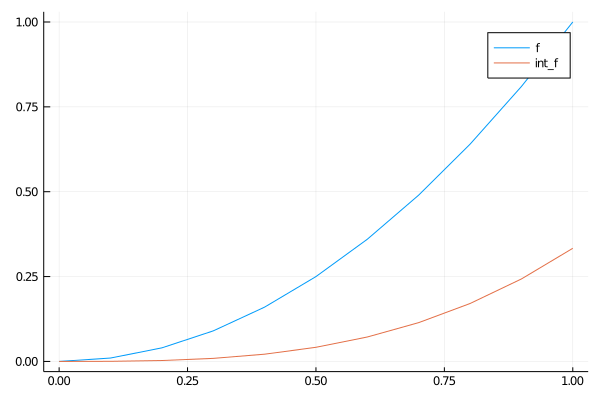Of course, univariate polynomials are another type of univariate function In : using Polynomials p = Polynomial([2, -5, 2], :x) # :x just gives a symbol for display @show p @show p(1.0) # call like a function plotfunctions(p) # same generic function  p = Polynomial(2 - 5*x + 2*x^2) p(1.0) = -1.0  Out: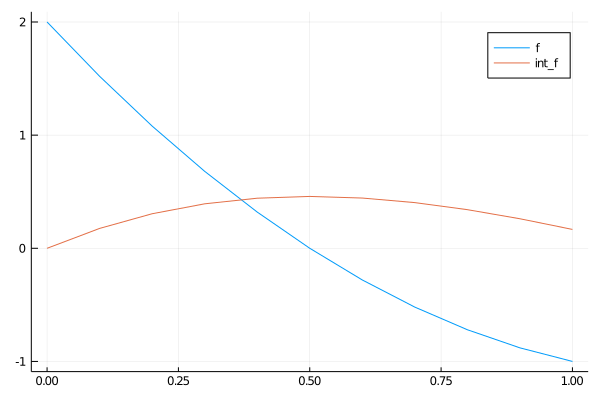Similarly, the result of interpolating data is also a function In : using Interpolations x = 0.0:0.2:1.0 f(x) = x^2 f_int = LinearInterpolation(x, f.(x)) # interpolates the coarse grid @show f_int(1.0) # call like a function plotfunctions(f_int) # same generic function  f_int(1.0) = 1.0  Out: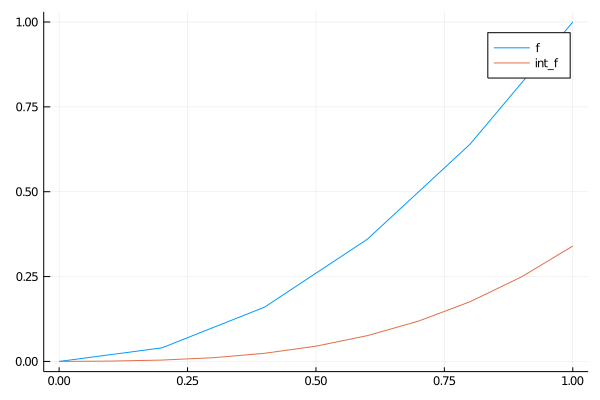Note that the same generic plotfunctions could use any variable passed to it that “looks” like a function, i.e., can call f(x). This approach to design with types – generic, but without any specific type declarations – is called duck typing. If you need to make an existing type callable, see Function Like Objects. ## Limitations of Dispatching on Abstract Types¶ You will notice that types in Julia represent a tree with Any at the root. The tree structure has worked well for the above examples, but it doesn’t allow us to associate multiple categorizations of types. For example, a semi-group type would be useful for a writing generic code (e.g. continuous-time solutions for ODEs and matrix-free methods), but cannot be implemented rigorously since the Matrix type is a semi-group as well as an AbstractArray, but not all semi-groups are AbstractArray s. The main way to implement this in a generic language is with a design approach called “traits”. ## Exercises¶ ### Exercise 1a¶ In a previous exercise, we discussed the trapezoidal rule for numerical integration. To summarize, the vector $$\int_{\underline{x}}^{\bar{x}} f(x) \, dx \approx \omega \cdot \vec{f}$$ where$ \vec{f} \equiv \begin{bmatrix} f(x_1) & \ldots & f(x_N) \end{bmatrix}\in R^N $and, for a uniform grid spacing of$ \Delta \$,

$$\omega \equiv \Delta \begin{bmatrix} \frac{1}{2} & 1 & \ldots & 1 & \frac{1}{2}\end{bmatrix} \in R^N$$

The quadrature rule can be implemented easily as

In :
using LinearAlgebra
function trap_weights(x)
return step(x) * [0.5; ones(length(x) - 2); 0.5]
end
x = range(0.0, 1.0, length = 100)
ω = trap_weights(x)
f(x) = x^2
dot(f.(x), ω)

Out:
0.3333503384008434

However, in this case the creation of the ω temporary is inefficient as there are no reasons to allocate an entire vector just to iterate through it with the dot. Instead, create an iterable by following the interface definition for Iteration, and implement the modified trap_weights and integration.

Hint: create a type such as

In :
struct UniformTrapezoidal
count::Int
Δ::Float64
end


and then implement the function Base.iterate(S::UniformTrapezoidal, state=1).

Make the UniformTrapezoidal type operate as an array with interface definition for AbstractArray. With this, you should be able it go ω or length(ω) to access the quadrature weights.